Published

# Automatic car parking system project Using Arduino

In this, we are making an automatic car parking system using Arduino and display the messages on 16x2 LCD.

IntermediateProtip2 hours131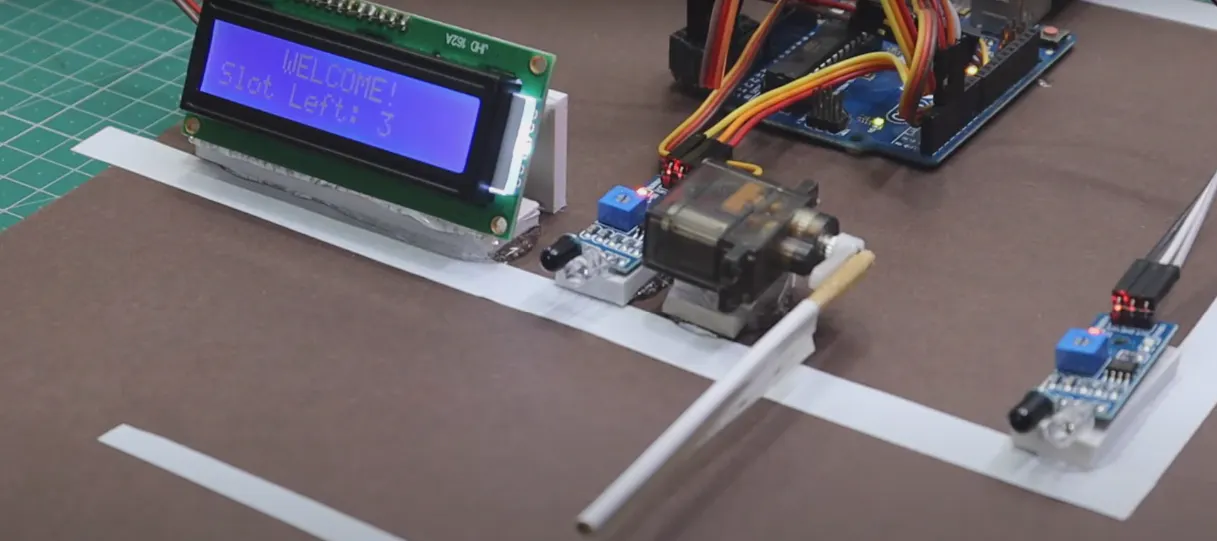## Things used in this project

### Hardware componentsArduino UNO
×1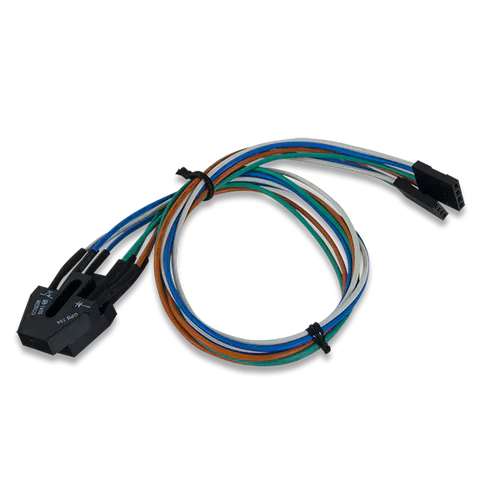Digilent IR Proximity Sensor
×2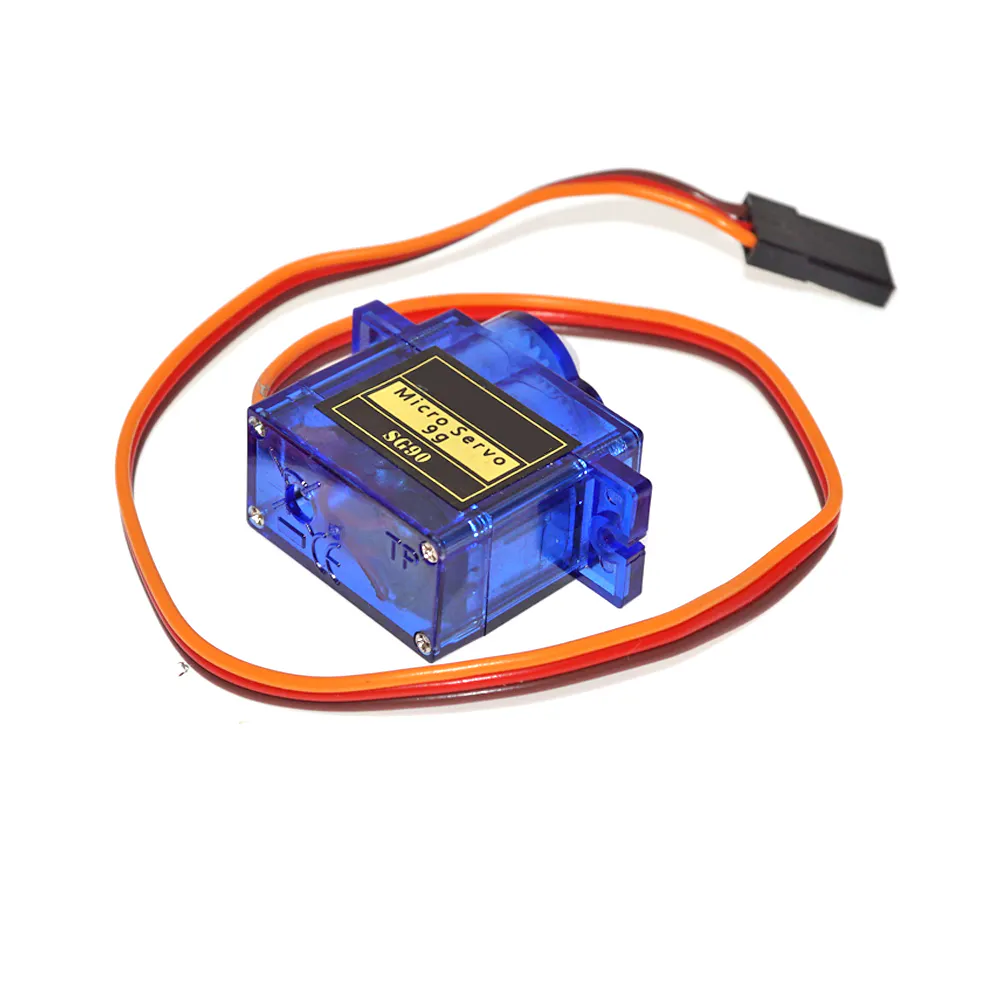SG90 Micro-servo motor
×1Jumper wires (generic)
×1×1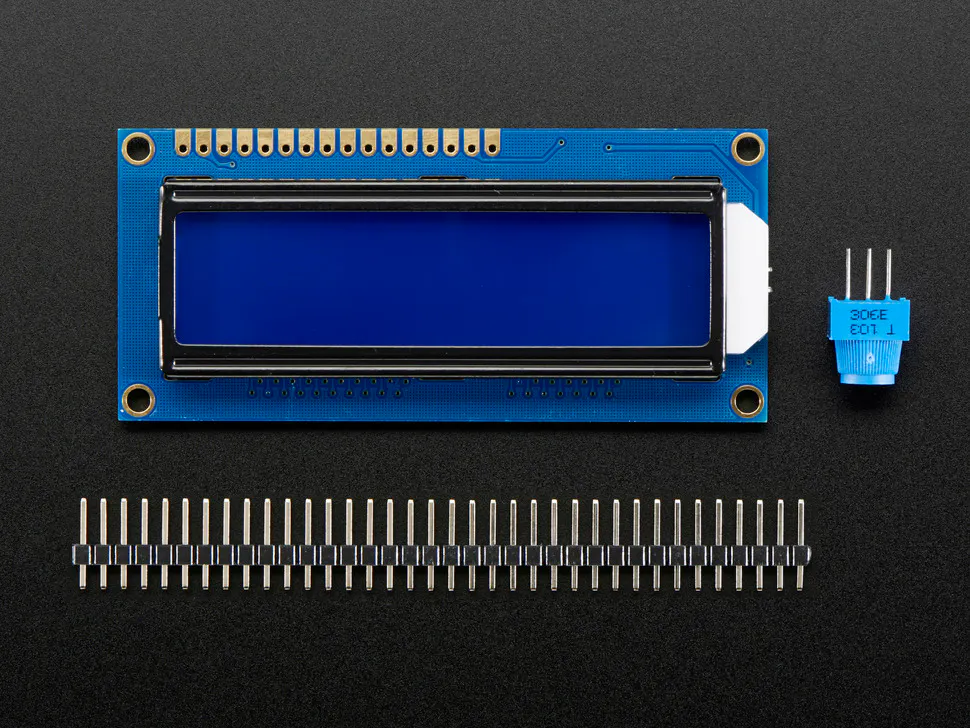Adafruit Standard LCD - 16x2 White on Blue
×1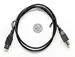USB-A to B Cable
×1

### Software apps and online servicesArduino IDE

## Code

### Code snippet #1

Plain text
``` // TECHATRONIC.COM
// I2C LIBRARY
//https://github.com/fdebrabander/Arduino-LiquidCrystal-I2C-library
#include <Wire.h>
#include <LiquidCrystal_I2C.h>
LiquidCrystal_I2C lcd(0x27,16,2);
#include <Servo.h>
Servo myservo1;
int IR1 = 4; // IR Sensor 1
int IR2 = 7; // IR Sensor 2
int Slot = 4;      //Enter Total number of parking Slots
int flag1 = 0;
int flag2 = 0;
void setup()
{
lcd.init();
lcd.backlight();
pinMode(IR1, INPUT);
pinMode(IR2, INPUT);
myservo1.attach(9);
myservo1.write(100);
lcd.setCursor (0,0);
lcd.print("   ARDUINO  ");
lcd.setCursor (0,1);
lcd.print(" PARKING SYSTEM ");
delay (2000);
lcd.clear();
}
void loop(){
if(digitalRead (IR1) == LOW && flag1==0){
if(Slot>0){flag1=1;
if(flag2==0){myservo1.write(0); Slot = Slot-1;}
}else{
lcd.setCursor (0,0);
lcd.print("  SORRY 🙁  ");
lcd.setCursor (0,1);
lcd.print(" Parking Full ");
delay (3000);
lcd.clear();
}
}
if(digitalRead (IR2) == LOW && flag2==0){flag2=1;
if(flag1==0){myservo1.write(0); Slot = Slot+1;}
}
if(flag1==1 && flag2==1){
delay (1000);
myservo1.write(100);
flag1=0, flag2=0;
}
lcd.setCursor (0,0);
lcd.print("  WELCOME!  ");
lcd.setCursor (0,1);
lcd.print("Slot Left: ");
lcd.print(Slot);
}
```

### Code snippet #2

Plain text
``` // TECHATRONIC.COM
// I2C LIBRARY
//https://github.com/fdebrabander/Arduino-LiquidCrystal-I2C-library
#include <Wire.h>
#include <LiquidCrystal_I2C.h>
LiquidCrystal_I2C lcd(0x27,16,2);
#include <Servo.h>
Servo myservo1;
int IR1 = 4; // IR Sensor 1
int IR2 = 7; // IR Sensor 2
int Slot = 4;      //Enter Total number of parking Slots
int flag1 = 0;
int flag2 = 0;
void setup()
{
lcd.init();
lcd.backlight();
pinMode(IR1, INPUT);
pinMode(IR2, INPUT);
myservo1.attach(9);
myservo1.write(100);
lcd.setCursor (0,0);
lcd.print("   ARDUINO  ");
lcd.setCursor (0,1);
lcd.print(" PARKING SYSTEM ");
delay (2000);
lcd.clear();
}
void loop(){
if(digitalRead (IR1) == LOW && flag1==0){
if(Slot>0){flag1=1;
if(flag2==0){myservo1.write(0); Slot = Slot-1;}
}else{
lcd.setCursor (0,0);
lcd.print("  SORRY 🙁  ");
lcd.setCursor (0,1);
lcd.print(" Parking Full ");
delay (3000);
lcd.clear();
}
}
if(digitalRead (IR2) == LOW && flag2==0){flag2=1;
if(flag1==0){myservo1.write(0); Slot = Slot+1;}
}
if(flag1==1 && flag2==1){
delay (1000);
myservo1.write(100);
flag1=0, flag2=0;
}
lcd.setCursor (0,0);
lcd.print("  WELCOME!  ");
lcd.setCursor (0,1);
lcd.print("Slot Left: ");
lcd.print(Slot);
}
```

### Github

https://github.com/fdebrabander/Arduino-LiquidCrystal-I2C-library

### Github

https://github.com/PaulStoffregen/Wire

## Credits

### Techatronic

57 projects • 46 followers
Electronic engineer